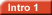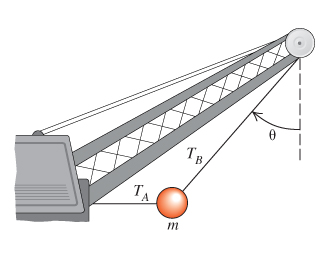# Problem: A large wrecking ball is held in place by two light steel cables.a)If the mass m of the wrecking ball is 4040 kg, what is the tension TB in the cable that makes an angle of 40.0 with the vertical?Take the free fall acceleration to be g = 9.80 m/s.b)If the mass m of the wrecking ball is 4040 kg, what is the tension TA in the horizontal cable?Take the free fall acceleration to be g = 9.80 m/s2.

🤓 Based on our data, we think this question is relevant for Professor Aparicio-Bolano's class at UM.

###### FREE Expert Solution

Basic trigonometry:

The weight of the ball, TA, and TB vectors make a right angle triangle such that:

TB is the hypotenuse.

TA is the opposite

(a)

cos θ = mg/TB###### Problem Details

A large wrecking ball is held in place by two light steel cables.a)

If the mass m of the wrecking ball is 4040 kg, what is the tension TB in the cable that makes an angle of 40.0with the vertical?

Take the free fall acceleration to be g = 9.80 m/s.

b)

If the mass m of the wrecking ball is 4040 kg, what is the tension TA in the horizontal cable?

Take the free fall acceleration to be g = 9.80 m/s2.